Precalculus : Graph a Polynomial Function

Example Questions

Example Question #3 : Graphs Of Polynomial Functions

Graph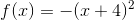.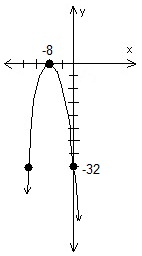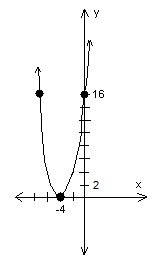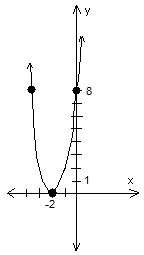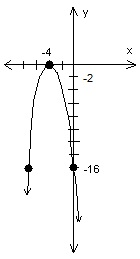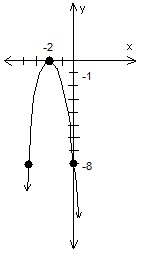Explanation:is a parabola, because of the general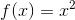structure.  The parabola opens downward because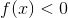.

Solving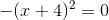tells the x-value of the x-axis intercept;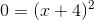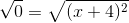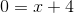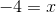The resulting x-axis intercept is: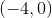.

Settingtells the y-value of the y-axis intercept;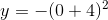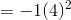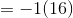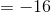The resulting y-axis intercept is: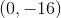All Precalculus Resources